Syntax Diagrams
A programming language is a set of rules, symbols, and special words used to construct a program. There are rules for both syntax (grammar) and semantics (meaning).

Syntax: The formal rules governing how one writes valid instructions in a language
Semantics: The set of rules that gives the meaning of instructions written in a programming language.

A useful tool for accurately describing syntax rule is the syntax diagram. The following is an example to explain how syntax diagrams work.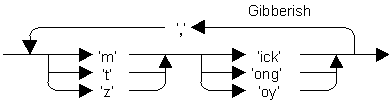This syntax diagram shows how to form a piece of gibberish. By following the arrows from entry to exit, we can construct a syntactically correct piece of gibberish such as:

• moy, toy, zick, zong, mick
There is no pattern for using the diagram other than beginning at the left and obeying the direction of the arrows. At a branching point you may go either way. At the first branch you could take an "m", 't', or 'z'. Then add 'ick', 'ong', or 'oy'. You could stop there, or you could add a ',' and then go back to the beginning and start again.

Another way of writing this diagram is as follows: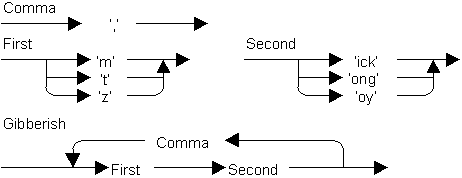Syntax diagrams are one way of writing algorithms. If you look closely at the gibberish diagrams, you will see all four of the basic structures of algorithms represented. There are sequences of instructions (first write and 'm', 't', or 'z', then write 'ick', 'ong', or 'oy'); there are selections (places where you can branch to different choices); and there are loops (writing a comma and going back to the start). The second way of writing gibberish diagrams involves subprograms (called Comma, First, and Second) and references to them. The two ways of writing the syntax diagrams demonstrate, once again, that there is more than one way to write any algorithm.

Recursion is employed in syntax diagrams. For example, in the C++ language an Identifier is defined as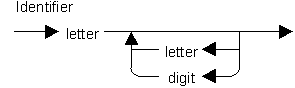Algebraic Expressions
Here is an incorrect expression where the right and left parentheses are equal in number, but something else is wrong.

• `a + ( b - c ) + d ) - e + ( x + ( -z )`
What principle could we use to reject this string of symbols?

In addition to problems with parentheses, there may be problems with the operators `+, -, *, /` as in the following expressions:

• `a + * b`
• `a + ( * b + c )`
It might be possible to make a complete list or errors that make the syntax of an algebraic expression incorrect. However a "Syntax diagram" is more useful.

In Algebraic expressions, a term is any numerical value such as 4, 5.7, or -0.0005 or a variable name standing for the same, then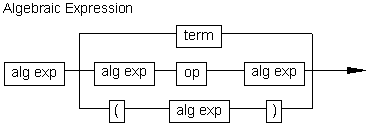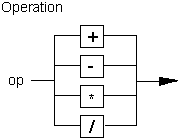Continue to:  Unit 3 / Prev / Next